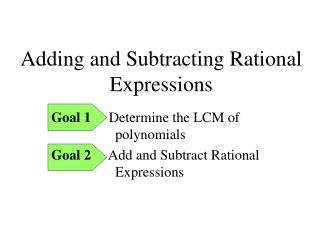DownloadDownload Presentation# Adding and Subtracting Rational Expressions

Download Presentation## Adding and Subtracting Rational Expressions

- - - - - - - - - - - - - - - - - - - - - - - - - - - E N D - - - - - - - - - - - - - - - - - - - - - - - - - - -
##### Presentation Transcript

1. Adding and Subtracting Rational Expressions Goal 1 Determine the LCM of polynomials Goal 2 Add and Subtract Rational Expressions

2. What is the Least Common Multiple? Fractions require you to find the Least Common Multiple (LCM) in order to add and subtract them! • Least Common Multiple (LCM) - smallestnumber or polynomial into which each of the numbers or polynomials will divide evenly. The Least Common Denominator is the LCM of the denominators.

3. Find the LCM of each set of Polynomials 1) 12y2, 6x2 LCM = 12x2y2 2) 16ab3, 5a2b2, 20ac LCM = 80a2b3c 3) x2 – 2x, x2 - 4 LCM = x(x + 2)(x – 2) 4) x2 – x – 20, x2 + 6x + 8 LCM = (x + 4) (x – 5) (x + 2)

4. Adding Fractions - A Review LCD is 12. Find equivalent fractions using the LCD. Collect the numerators, keeping the LCD.

5. Remember: When adding or subtracting fractions, you need a common denominator! When Multiplying or Dividing Fractions, you don’t need a common Denominator

6. Steps for Adding and Subtracting Rational Expressions: 1. Factor, if necessary. 2. Cancel common factors, if possible. 3. Look at the denominator. 4. Reduce, if possible. 5. Leave the denominators in factored form. If the denominators are the same, add or subtract the numerators and place the result over the common denominator. If the denominators are different, find the LCD. Change the expressions according to the LCD and add or subtract numerators. Place the result over the common denominator.

7. Addition and Subtraction • Is the denominator the same?? • Example: Simplify Find the LCD: 6x Now, rewrite the expression using the LCD of 6x Simplify... Add the fractions... =19 6x

8. Simplify: Example 1 + 8(5n) - 7(15m) 6(3mn2) Multiply by 5n LCD = 15m2n2 m ≠ 0 n ≠ 0 Multiply by 15m Multiply by 3mn2

9. Examples: Example 2

10. Simplify: Example 3 (3x + 2) - (2x) (15) - (4x + 1) (3) (5) LCD = 15 Mult by 15 Mult by 3 Mult by 5

11. Simplify: Example 4

12. Simplify: Example 5 - (2b) a ≠ 0 b ≠ 0 (4a) (a) (b) LCD = 3ab

13. Adding and Subtracting with polynomials as denominators Simplify: Find the LCD: (x + 2)(x – 2) Rewrite the expression using the LCD of (x + 2)(x – 2) Simplify... – 5x – 22 (x + 2)(x – 2)

14. Adding and Subtracting with Binomial Denominators (x + 3) (x + 1) 2 + 3 Multiply by (x + 3) Multiply by (x + 1) LCD = (x + 3)(x + 1) x ≠ -1, -3

15. Simplify: Example 6 ** Needs a common denominator 1st! Sometimes it helps to factor the denominators to make it easier to find your LCD. LCD: 3x3(2x+1)

16. Simplify: Example 7 LCD: (x+3)2(x-3)

17. Simplify: Example 8 (x + 2) - 3x (x - 1) 2x x ≠ 1, -2

18. Simplify: Example 9 3x (x - 1) - 2x (x + 2) (x + 3)(x + 2) (x + 3)(x - 1) LCD (x + 3)(x + 2)(x - 1) x ≠ -3, -2, 1

19. Simplify: Example 10 4x + 5x (x - 3) (x - 2) (x - 3)(x - 2) (x - 2)(x - 2) LCD (x - 3)(x - 2)(x - 2) x ≠ 3, 2

20. Simplify: Example 11 (x - 2) (x + 1) (x + 3) - (x - 4) (x - 1)(x + 1) (x - 2)(x - 1) LCD (x - 1)(x + 1)(x - 2) x ≠ 1, -1, 2

21. Pg 173 # 1 – 21 odd